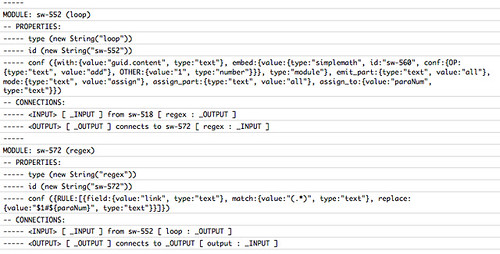# The Yahoo Pipes Documentation Project – Initial Thoughts

One of the issues I keep coming across when trying to get folk interested in using Yahoo Pipes is the question of what happens if Yahoo Pipes Dies? I tend to post a lot of screenshots from some of the pipes I build, so I’d stand some chance of recreating them elsewhere, but this is not generally the case for most pipes users.

If we could compile a version of pipe into code that could be run on our own servers, then we could use Yahoo Pipes as a rapid prototyping tool and code generator, rather than as a runtime platfrom. This might then make the environment more palatable to developers working in a conservative regime (e.g. Starting to Think About a Yahoo Pipes Code Generator).

As a stop gap, I thought it would be worth starting up a little Yahoo Pipes Documentation Project to produce some sort of documentation around Yahoo pipes. Grabbing JSON Data from One Web Page and Displaying it in Another contains a couple of bookmarks that demonstrate a simple way of grabbing the content of a pipe.

Here’s a first attempt reporter, done as a bookmarklet that can be applied when editing a pipe. The result is dsiplayed in the browser console:

`javascript:(function(){var p=editor.pipe.working;var d="----- ";var c=console.log;c(d+d);var q,r,w,i,m,k,K,ok,oK,x,X;var D=false;var ins=[];var outs=[];var b=[];var o=[];if(D)c("CONNECTIONS");m=p.wires;for (i=0;i<m.length;i++){if(D)c(d);q=m[i].src;r=m[i].tgt;K=r.moduleid;k=q.moduleid;if (!(b[K])){b[K]={};b[K].conns=[]};if (!(b[k])){b[k]={};b[k].conns=[]};ok={};oK={};x=b[k].conns.length;X=b[K].conns.length;b[k].conns[x]={};b[k].conns[x].o=q.id;b[k].conns[x].typ="out";b[k].conns[x].t=K;b[k].conns[x].T=r.id;b[K].conns[X]={};b[K].conns[X].typ="in";b[K].conns[X].f=k;b[K].conns[X].F=q.id;b[K].conns[X].i=r.id;if(D)c("FROM "+k+" ("+q.id+") TO "+K+" ("+r.id+")");}if(D)c(d+d); m=p.modules;c('MODULES');for (i=0;i<m.length;i++){q=m[i];if (!(b[q.id]))b[q.id]=[];b[q.id].n=q.type};for (i=0;i < m.length;i++){c(d);q=m[i];k=q.id;c("MODULE: "+k+" ("+q.type+")");c("-- PROPERTIES:");for (w in q)c(d+w+" "+q[w].toSource()+" ");c("-- CONNECTIONS:");if (b[k])if(b[k].conns)for (j=0;j<b[k].conns.length;j++){K=b[k].conns[j];if(K.typ=="in"){w=d+'<INPUT> [ '+K.i+' ]';if (K.f)w+=' from '+K.f+' [ '+b[K.f].n+" : "+K.F+' ]';c(w)};if(K.typ=="out")c(d+'<OUTPUT> [ '+K.o+' ] connects to '+K.t+' [ '+b[K.t].n+' : '+K.T+' ]')}};c(d+d)})()`

Here’s a snippet of the sort of display it gives:PS @hapdaniel just alerted me to a way I can get hold of a description of a pipe and make it available via a JSON feed. I’ll get a post up about how to do that when I get a chance… Note that this approach deprecates the method above and the approach alluded to in Grabbing JSON data post. (In the meantime, here’s a taster…)

## Author: Tony Hirst

I'm a Senior Lecturer at The Open University, with an interest in #opendata policy and practice, as well as general web tinkering...